## 【小白学PyTorch】6 模型的构建访问遍历存储（附代码）

• 模型构建的函数：`add_module`,`add_module`,`add_module`
• 访问子模块：`add_module`,`add_module`,`add_module``add_module`
• 网络遍历：
`add_module`,`add_module`
• 模型的保存与加载：`add_module`,`add_module`,`add_module`

## 1 模型构建函数

`torch.nn.Module`是所有网络的基类，在PyTorch实现模型的类中都要继承这个类（这个在之前的课程中已经提到）。在构建Module中，Module是一个包含其他的Module的，类似于，你可以先定义一个小的网络模块，然后把这个小模块作为另外一个网络的组件。因此网络结构是呈现树状结构

``````import torch.nn as nn
import torch
class MyNet(nn.Module):
def __init__(self):
super(MyNet,self).__init__()
self.conv1 = nn.Conv2d(3,64,3)
self.conv2 = nn.Conv2d(64,64,3)

def forward(self,x):
x = self.conv1(x)
x = self.conv2(x)
return x
net = MyNet()
print(net)
``````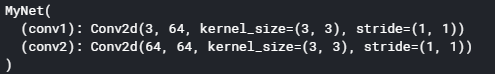`MyNet`中有两个属性`conv1``conv2`是两个卷积层，在正向传播`forward`的过程中，依次调用这两个卷积层实现网络的功能。

``````class MyNet(nn.Module):
def __init__(self):
super(MyNet,self).__init__()

def forward(self,x):
x = self.conv1(x)
x = self.conv2(x)
return x
``````

### 1.2 ModuleList

`ModuleList`按照字面意思是用`list`的形式保存网络层的。这样就可以先将网络需要的layer构建好，保存到一个list，然后通过`ModuleList`方法添加到网络中.

``````class MyNet(nn.Module):
def __init__(self):
super(MyNet,self).__init__()
self.linears = nn.ModuleList(
[nn.Linear(10,10) for i in range(5)]
)

def forward(self,x):
for l in self.linears:
x = l(x)
return x
net = MyNet()
print(net)
``````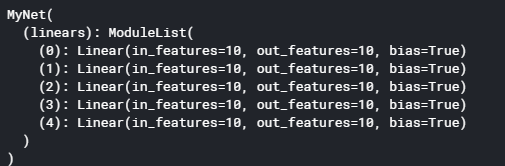``````vgg_cfg = [64, 64, 'M', 128, 128, 'M', 256, 256, 256, 'C', 512, 512, 512, 'M',
512, 512, 512, 'M']

def vgg(cfg, i, batch_norm=False):
layers = []
in_channels = i
for v in cfg:
if v == 'M':
layers += [nn.MaxPool2d(kernel_size=2, stride=2)]
elif v == 'C':
layers += [nn.MaxPool2d(kernel_size=2, stride=2, ceil_mode=True)]
else:
conv2d = nn.Conv2d(in_channels, v, kernel_size=3, padding=1)
if batch_norm:
layers += [conv2d, nn.BatchNorm2d(v), nn.ReLU(inplace=True)]
else:
layers += [conv2d, nn.ReLU(inplace=True)]
in_channels = v
return layers

class Model1(nn.Module):
def __init__(self):
super(Model1,self).__init__()

self.vgg = nn.ModuleList(vgg(vgg_cfg,3))

def forward(self,x):

for l in self.vgg:
x = l(x)
m1 = Model1()
print(m1)
``````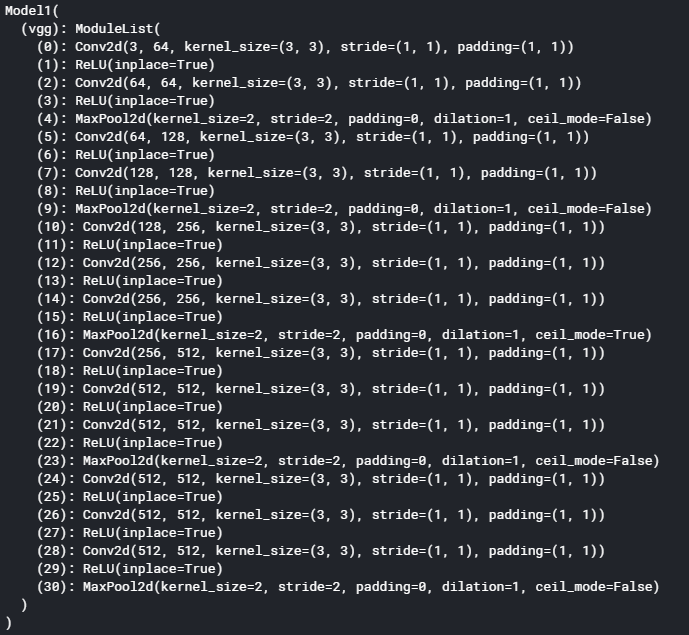### 1.3 Sequential

``````class MyNet(nn.Module):
def __init__(self):
super(MyNet,self).__init__()
self.conv = nn.Sequential(
nn.Conv2d(3,64,3),
nn.Conv2d(64,64,3)
)

def forward(self,x):
x = self.conv(x)
return x
net = MyNet()
print(net)
``````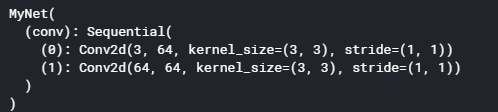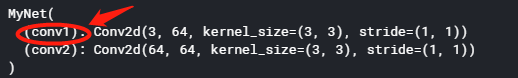``````from collections import OrderedDict
class MyNet(nn.Module):
def __init__(self):
super(MyNet,self).__init__()
self.conv = nn.Sequential(OrderedDict([
('conv1',nn.Conv2d(3,64,3)),
('conv2',nn.Conv2d(64,64,3))
]))

def forward(self,x):
x = self.conv(x)
return x
net = MyNet()
print(net)
``````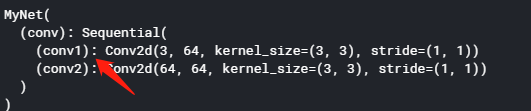### 1.4 小总结

• 单独增加一个网络层或者子模块，可以用`add_module`或者直接赋予属性；
• `ModuleList`可以将一个Module的List增加到网络中，自由度较高。
• `Sequential`按照顺序产生一个Module模块。这里推荐习惯使用OrderedDict的方法进行构建。对网络层加上规范的名称，这样有助于后续查找与遍历

## 2 遍历模型结构

``````import torch.nn as nn
import torch
from collections import OrderedDict
class MyNet(nn.Module):
def __init__(self):
super(MyNet,self).__init__()
self.conv1 = nn.Conv2d(in_channels=3,out_channels=64,kernel_size=3)
self.conv2 = nn.Conv2d(64,64,3)
self.maxpool1 = nn.MaxPool2d(2,2)

self.features = nn.Sequential(OrderedDict([
('conv3', nn.Conv2d(64,128,3)),
('conv4', nn.Conv2d(128,128,3)),
('relu1', nn.ReLU())
]))

def forward(self,x):
x = self.conv1(x)
x = self.conv2(x)
x = self.maxpool1(x)
x = self.features(x)

return x
net = MyNet()
print(net)
``````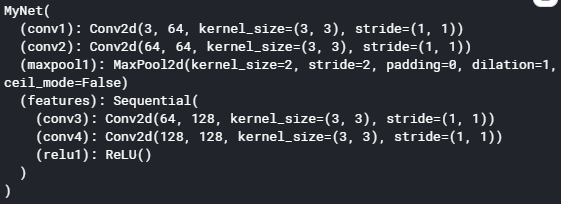### 2.1 modules()

``````for idx,m in enumerate(net.modules()):
print(idx,"-",m)
``````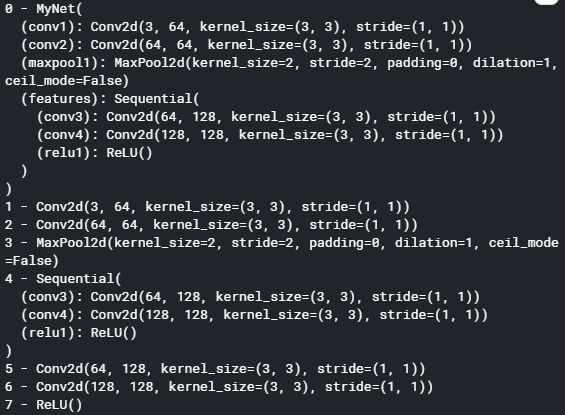• 首先第一个输出的是最大的那个Module，也就是整个网络，`0-Model`整个网络模块；
• `1-2-3-4`是网络的四个子模块，`4-Sequential`中间仍然包含子模块
• `5-6-7`是模块`4-Sequential`的子模块。

【总结】

`modules()`是递归的返回网络的各个module（深度遍历），从最顶层直到最后的叶子的module。

### 2.2 named_modules()

`named_modules()``module()`类似，只是同时返回name和module。

``````for idx,(name,m) in enumerate(net.named_modules()):
print(idx,"-",name)
``````### 2.3 parameters()

``````for p in net.parameters():
print(type(p.data),p.size())
``````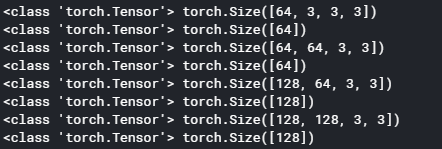``````optimizer = torch.optim.SGD(net.parameters(),
lr = 0.001,
momentum=0.9)
``````

``````for idx,(name,m) in enumerate(net.named_parameters()):
print(idx,"-",name,m.size())
``````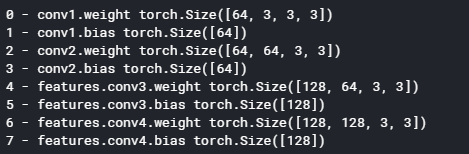【小扩展】

``````for idx,(name,m) in enumerate(net.named_modules()):
if isinstance(m,nn.Conv2d):
print(m.weight.shape)
print(m.bias.shape)
``````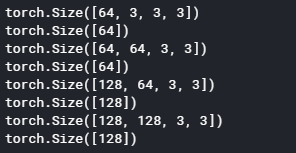## 3 保存与载入

PyTorch使用`torch.save``torch.load`方法来保存和加载网络，而且网络结构和参数可以分开的保存和加载。

``````torch.save(model,'model.pth') # 保存
``````

pytorch中网络结构和模型参数是可以分开保存的。上面的方法是两者同时保存到了.pth文件中，当然，你也可以仅仅保存网络的参数来减小存储文件的大小。注意：如果你仅仅保存模型参数，那么在载入的时候，是需要通过运行代码来初始化模型的结构的。

``````torch.save(model.state_dict(),"model.pth") # 保存参数
model = MyNet() # 代码中创建网络结构
``````

## 发布时间：2020-9-5 18:53 Saturday版权声明：除非注明，文章均为【舞乂IT-专注于网络技术分享】原创，欢迎转载！转载请注明本文地址，谢谢！